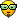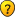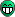Support

If you have a problem or need to report a bug please email : support@dsprobotics.com

There are 3 sections to this support area:

HELP & INFORMATION: tutorials and example files for learning or finding pre-made modules for your projects

USER FORUMS: meet with other users and exchange ideas, you can also get help and assistance here

## Impulse Response

DSP related issues, mathematics, processing and techniques

### Impulse Response

I wanted to understand the algorithmic of it. I learned that you have to transform to frequency domain using FFT, then calculate both and bring the result back to time domain using iFFT.
As far as I know, Flowstone is too slow at realtime FFT'ing, so I about other approaches. Unfortunately I learned that I already fail at the very beginning.

Basically, each sample of a signal has to be calculated with each sample of the impulse response. But how? I couldn't find any info on this (probably because it is so heavy on the CPU that nobody bothers to even think about it). But I need to understand the relationship of both signals. Here are 3 calculations I can think of:

Code: Select all
`Given thatSignal: 0.5Impulse Response: 1, 0.5, 0.250.5 * 1 = 0.5              * 0.5 = 0.25                           * 0.25 = 0.06250.5 + 1 + 0.5 + 0.25 = 2.25                            / 3 = 0.75                                          (0.5 + 1) / 2 = 0.75                     + 0.5) / 2 = 0.625                                        + 0.25) / 2 = 0.4375`

All other possibilities would lead to a signal raising far beyond the normalized range of [-1, 1]. So how would the input signal 0.5 be calculated with the impulse response [1, 0.5, 0.25] ?
tulamide

Posts: 1931
Joined: Sat Jun 21, 2014 2:48 pm
Location: Germany

### Re: Impulse Response

Not sure about that, but there are two ways to apply IR to a signal:

1. in Frequency Domain
1. Get IR
2a. If IR is in time Domain -> FFT it
2b. If IR is in freq Domain -> iFFT it (=smoothing), then FFT it again
4. Complex multiply your input with the IR (as they are both FFT with Real/Imag parts)
5. iFFT the result
An example for this you can actually find in the StreamFFT project:
viewtopic.php?f=4&t=1510&start=60#p6660

2. in Time Domain
1. Get IR
2a. If IR is in time Domain -> FFT it
2b. If IR is in freq Domain -> iFFT it (=smoothing), then FFT it again
3. Use IR FFT as Input to a FIR of the same size then run input through it
An example can be found here:
http://dsprobotics.com/support/viewtopi ... t=10#p6877

The math behind it isn't that trivial, but well documented and in both cases it boils down to convolution... which is basically mulitplying 2 arrays together and you get another array... then take the sum of each of its elements. Like this in pseudo code:
Code: Select all
`array X[length]array Y[length]output = 0for i = 0 to length-1   output = output + X[i]*Y[i]`MyCo

Posts: 764
Joined: Tue Jul 13, 2010 12:33 pm
Location: Germany

### Re: Impulse Response

Thanks, MyCo. I'm currently reading both threads, but it is "Böhmische Dörfer" to me.

May I pass your attention to this explanation of "Faltungshall" in german? (All non-germans please excuse): Faltungshall
In the paragraph "Faltung" I read the following sentences:
"Rein theoretisch wäre die Multiplikation der Frequenzbilder im Frequenzraum nicht nötig. Man könnte stattdessen jeden Zeitpunkt des zu verhallenden Signals mit jedem Zeitpunkt der Impulsantwort multiplizieren. Die Rechenmethode dafür heißt Faltung:"My problem is that I don't understand the formulatulamide

Posts: 1931
Joined: Sat Jun 21, 2014 2:48 pm
Location: Germany

### Re: Impulse Response

The idea behind a convolution reverb is the following:
1. The acoustic properties of a room may be characterised by its impulse response (IR).
2. A sound source signal may be viewed as a sequence of impulses (samples).
3. The room response to a sound source may be viewed as the sum of (overlapping) responses to each sample.

The last step is what mathematicians call convolution (German: Faltung). In your formula, f(t) represents the source signal and g(t) denotes the IR. The left hand side of the equation is the reverbed signal at time t. The right hand side is the sum (or integral in the continuous time domain) over all previous samples at time tau that excited a response, which took some time (t - tau) to finally reach the listener's ear at time t.

The concept is fairly simple, however its implementation requires some additional brainwork. Think about it: each sample of the dry signal produces a reverb tail in the range of seconds (many thousands of samples). Hence, to add reverb, you would have to sum (for each reverbed sample!) that huge number of overlapping tails. It is not viable to do that via brute force.

That's where FFT comes in. FFT can do convolutions at a fraction of the CPU load needed for brute force convolution. However, that's not the whole story, because plain vanilla FFT introduces latency of at least the window size. There are algorithms to deal with that issue, but that's where it really gets really, really tricky.martinvicanek

Posts: 915
Joined: Sat Jun 22, 2013 8:28 pm

### Re: Impulse Response

Regarding the wikipedia article:
Ignore any formula with an integral. Integrals are useless for any DSP processing, that's why they also give a discrete solution:When you look a bit closer you might see some similarities to the pseudo code that I posted:
Code: Select all
`// length is "N"array X[length]  // this is "x" from x(i) to x(i - (N-1))array Y[length]  // this is "h"output = 0     // this is "y(i)"for i = 0 to length-1 // i is "j"   output = output + X[i]*Y[i]`

When you convert the formula directly into usable pseudo code you need some kind of ringbuffer to store older input samples so you can use them (for the x(i-j) part), this can look like this:
Code: Select all
`array x[N] // ringbuffer nowarray h[N] shift xi into x[N] from left // xi is the current input sample// so x is xi now, and the old x[N-1] is droppedyi = 0 // outputfor j = 0 to N-1   yi = yi + x[i]*h[i]`

That's a very crude way of doing it. A better way to do that is actually in the FIR example I linked above, it uses a write pointer into the array, so it's a true ringbufferMyCo

Posts: 764
Joined: Tue Jul 13, 2010 12:33 pm
Location: Germany

### Re: Impulse Response

martinvicanek wrote:The idea behind a convolution reverb is the following:
1. The acoustic properties of a room may be characterised by its impulse response (IR).
2. A sound source signal may be viewed as a sequence of impulses (samples).
3. The room response to a sound source may be viewed as the sum of (overlapping) responses to each sample

Yes, I figured out 1 and 2. For 2 I used this very beginner friendly document: http://www.ling.upenn.edu/courses/cogs501/ImpulseResponse.html. But halfway down that document I got lost.
3 is what I'm struggling with. I know all the theory behind it, but when it comes to actual code I'm lost.

martinvicanek wrote:In your formula, f(t) represents the source signal and g(t) denotes the IR. The left hand side of the equation is the reverbed signal at time t. The right hand side is the sum (or integral in the continuous time domain) over all previous samples at time tau that excited a response, which took some time (t - tau) to finally reach the listener's ear at time t.

Now that is something I can understand! Why do mathematicians always hide behind obscure signs, instead of explaining it like you did? Numberphile shows me again and again that it must not be that hiding way, it can be explained in normal language! (Isn't it a shame that I had Mathe-Leistungskurs?)

martinvicanek wrote:That's where FFT comes in. FFT can do convolutions at a fraction of the CPU load needed for brute force convolution. However, that's not the whole story, because plain vanilla FFT introduces latency of at least the window size. There are algorithms to deal with that issue, but that's where it really gets really, really tricky.I wouldn't mind the latency. The very good ReaVerb, a VST from Cockos that comes bundled with their DAW Reaper, Introduces several second long latency, but you only notice it when switching it off (you will hear then the track with ReaVerb being out of sync to the other tracks for the duration of the buffer). In fact, Reaper has a so called "vst plugin latency compensation" and I assume that all DAWs have it?
The harder part is the fft'ing and the convolution. With the stream fft that MyCo linked to, would I be able to run both signals through 2 seperate FFTs? How to convolve the two results then? And how to make sure that the correct portion of the IR signal is used at any time? Is it just looped-playing of the IR waveform? So many questions...MyCo wrote:Ignore any formula with an integral. Integrals are useless for any DSP processing

I like short rules. I can remember them even without knowledge of the coherences. Goes straight into my mind!

MyCo wrote:Way easier to read. The Euler-like E means sum, so sum of all values from j == 0 to j == N - 1. I would have had a hard time figuring out N, so thank you for giving the pseudo code. I don't know why, but I can comprehend everything so much easier in code-form, even if it is in a code I never learned before (like I never had anything to do with Ruby, before learning it 18 months or so ago), or in pseudo-code.

MyCo wrote:When you convert the formula directly into usable pseudo code you need some kind of ringbuffer to store older input samples so you can use them (for the x(i-j) part), this can look like this:
Code: Select all
`array x[N] // ringbuffer nowarray h[N] shift xi into x[N] from left // xi is the current input sample// so x is xi now, and the old x[N-1] is droppedyi = 0 // outputfor j = 0 to N-1   yi = yi + x[i]*h[i]`

That's a very crude way of doing it. A better way to do that is actually in the FIR example I linked above, it uses a write pointer into the array, so it's a true ringbuffer

I can also see from the formula and your pseudo-code, that this is dependent on a certain range. In some convolution reverbs I know of there is a limitation of the IR length. Mostly 4 to 6 seconds. Since that length is N and the cpu load grows with N, it seems that they use something very similar to this.
tulamide

Posts: 1931
Joined: Sat Jun 21, 2014 2:48 pm
Location: Germany

### Re: Impulse Response

tulamide wrote:Why do mathematicians always hide behind obscure signs, instead of explaining it like you did? Numberphile shows me again and again that it must not be that hiding way, it can be explained in normal language.
Mathematicians have developed their own language mainly to express things in a compact, precise way. Without that sort of efficiency, they could hardly ever come up with something but trivial. Of course when talking to the rest of the world, they better use common terminology else nobody will understand and they will be perceived as snobs. (Some of them really are).
tulamide wrote:I wouldn't mind the latency. The very good ReaVerb, a VST from Cockos that comes bundled with their DAW Reaper, Introduces several second long latency, but you only notice it when switching it off (you will hear then the track with ReaVerb being out of sync to the other tracks for the duration of the buffer). In fact, Reaper has a so called "vst plugin latency compensation" and I assume that all DAWs have it?
Latency compensation is OK for offline studio work. It has yet to be invented for a live act, though.martinvicanek

Posts: 915
Joined: Sat Jun 22, 2013 8:28 pm

### Re: Impulse Response

martinvicanek wrote:Latency compensation is OK for offline studio work. It has yet to be invented for a live act, though.It is my strong belief that mankind is on the verge of inventing the time machine (actually we did it already - just one neutron/proton or so, but every walk starts with a little step, right? Now imagine you would outsource calculations to the future - instant results to everything! There's just the philosophical question if such a gig would be a live act, if the effects are calculated in the future?tulamide

Posts: 1931
Joined: Sat Jun 21, 2014 2:48 pm
Location: Germany

### Re: Impulse Response

tulamide wrote:The harder part is the fft'ing and the convolution. With the stream fft that MyCo linked to, would I be able to run both signals through 2 seperate FFTs? How to convolve the two results then? And how to make sure that the correct portion of the IR signal is used at any time? Is it just looped-playing of the IR waveform? So many questions...Now that I had time to think about it, there's a huge mistake in my thinking. Let me see if I am now right: FFT'ing a signal means to transfer it from the time domain to the frequency domain. In the frequency domain, the time is not a factor anymore. So it would be sufficient to fft the IR once, and then use the resulting data for all the incoming fft of the stream (the dry signal that shall be convolved). Is this right?

Now the convolving. How is it to be done? Say I have the following simplified arrays (already fft'd, so frequency domain):
Code: Select all
`SIGNAL 0.1, 0.2, 0.3, 0.4, 0.5IR     1.0, 0.9, 0.8, 0.7, 0.6`

Is it then
Code: Select all
`TYPE A0.1 * 1.0, 0.2 * 0.9, 0.3 * 0.8, 0.4 * 0.7, 0.5 * 0.6`

or
Code: Select all
`TYPE B0.1 * 1.0 * 0.9 * 0.8 * 0.7 * 0.60.2 * 1.0 * 0.9 * 0.8 * 0.7 * 0.60.3 * 1.0 * 0.9 * 0.8 * 0.7 * 0.60.4 * 1.0 * 0.9 * 0.8 * 0.7 * 0.60.5 * 1.0 * 0.9 * 0.8 * 0.7 * 0.6`

Also, what happens if for type b any of the IR data is 0? Multiplying by 0 results in 0, so all subsequent multiplications would also result in 0:

Code: Select all
`SIGNAL 0.1, 0.2, 0.3, 0.4, 0.5IR     1.0, 0.9, 0.0, 0.7, 0.60.1 * 1.0 * 0.9 * 0.0 * 0.7 * 0.6 = 00.2 * 1.0 * 0.9 * 0.0 * 0.7 * 0.6 = 00.3 * 1.0 * 0.9 * 0.0 * 0.7 * 0.6 = 00.4 * 1.0 * 0.9 * 0.0 * 0.7 * 0.6 = 00.5 * 1.0 * 0.9 * 0.0 * 0.7 * 0.6 = 0`

EDIT: I found a document from the WebAudio API that explains how it implements a convolution reverb. Maybe this is of interest for the DSP experts among us? I really hope to see some comments, tips, help, or even discouraging if this is something Flowstone can't handle.
https://dvcs.w3.org/hg/audio/raw-file/tip/webaudio/convolution.html
tulamide

Posts: 1931
Joined: Sat Jun 21, 2014 2:48 pm
Location: Germany

### Re: Impulse Response

tulamide wrote:Is it then
Code: Select all
`TYPE A0.1 * 1.0, 0.2 * 0.9, 0.3 * 0.8, 0.4 * 0.7, 0.5 * 0.6`

Yes and no! Each element gets multiplied by the corresponding element of the other array. But this is not a scalar multiplication like you showed, it's a complex one. You have to understand that an FFT yields 2 values for each frequency bin (real and imaginary part), using those 2 values you can get the Amplitude and the Phase of a frequency (those are just magnitude and angle of the complex number). So at the end you get:
Code: Select all
`res = (0.1+0.5i) * (1.0+0.2i)`

Complex multiplication would be like that:
Code: Select all
`(0.1*1.0 - 0.5*0.2) + (0.1*0.2 + 0.5*1.0)i`

Or for programmers:
Code: Select all
`res_real = (0.1*1.0 - 0.5*0.2) res_imag = (0.1*0.2 + 0.5*1.0)`

tulamide wrote:I really hope to see some comments, tips, help, or even discouraging if this is something Flowstone can't handle.

OK, just took a very short look... and it seems to be just interlacing of FFTs as we already do in the StreamFFT project. Basically they split the IR into multiple FFTs and store the previous FFTs of the input signal at the end they are doing a convolution on all of the FFTs they have... interesting but nothing I wouldn't expect. Could be done using StreamFFT... but it'll need a huge bunch of code.MyCo

Posts: 764
Joined: Tue Jul 13, 2010 12:33 pm
Location: Germany

Next